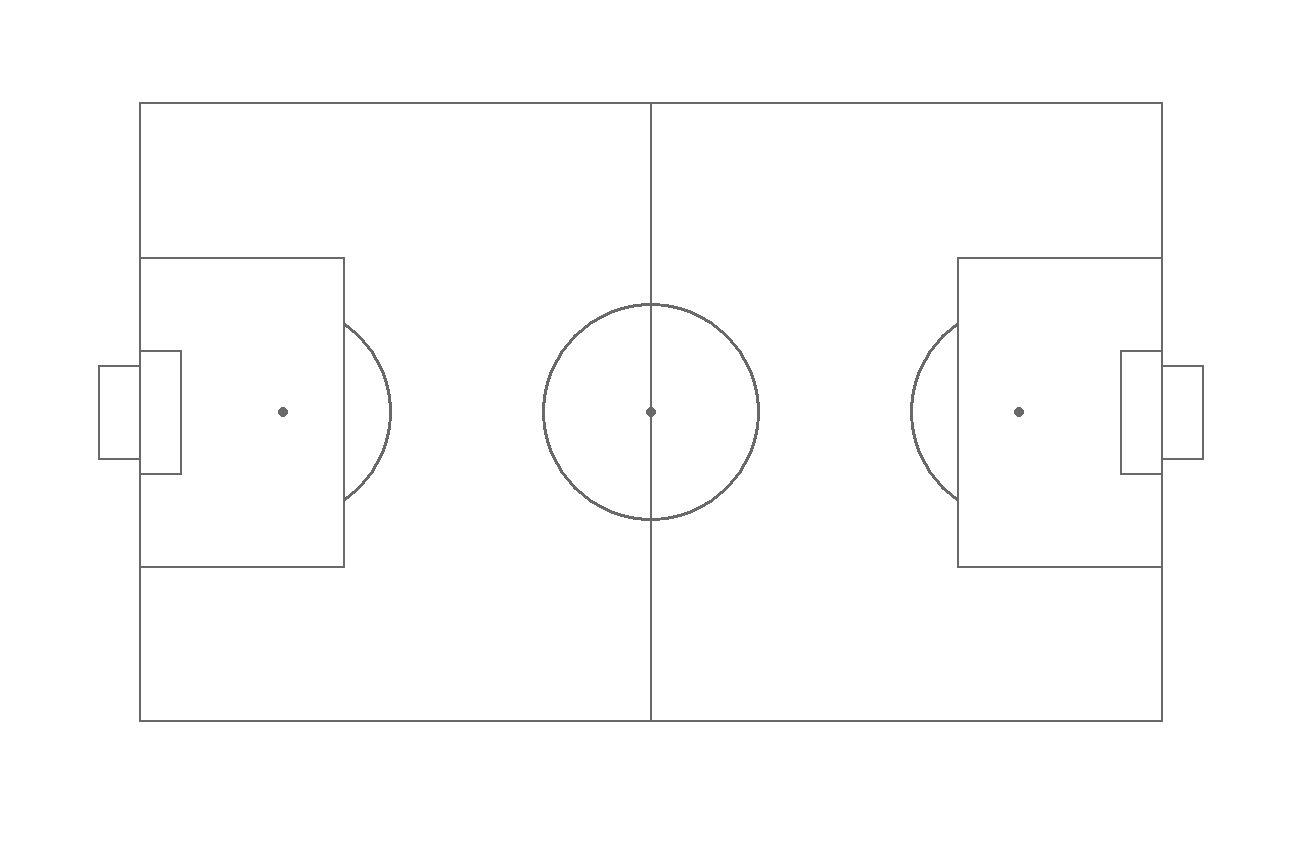# Soccer event data in ggplot2 with ggsoccer

Ben Torvaney

## Basic soccer pitch

Creating a soccer pitch with `ggsoccer` is straightforward. Load the library and add the `annotate_pitch` layer to `ggplot` function. In addition, you can use `theme_pitch` if you want a void theme.

``````# install.packages("ggplot2)")
# install.packages("ggsoccer")
library(ggplot2)
library(ggsoccer)

ggplot() +
annotate_pitch() +
theme_pitch()``````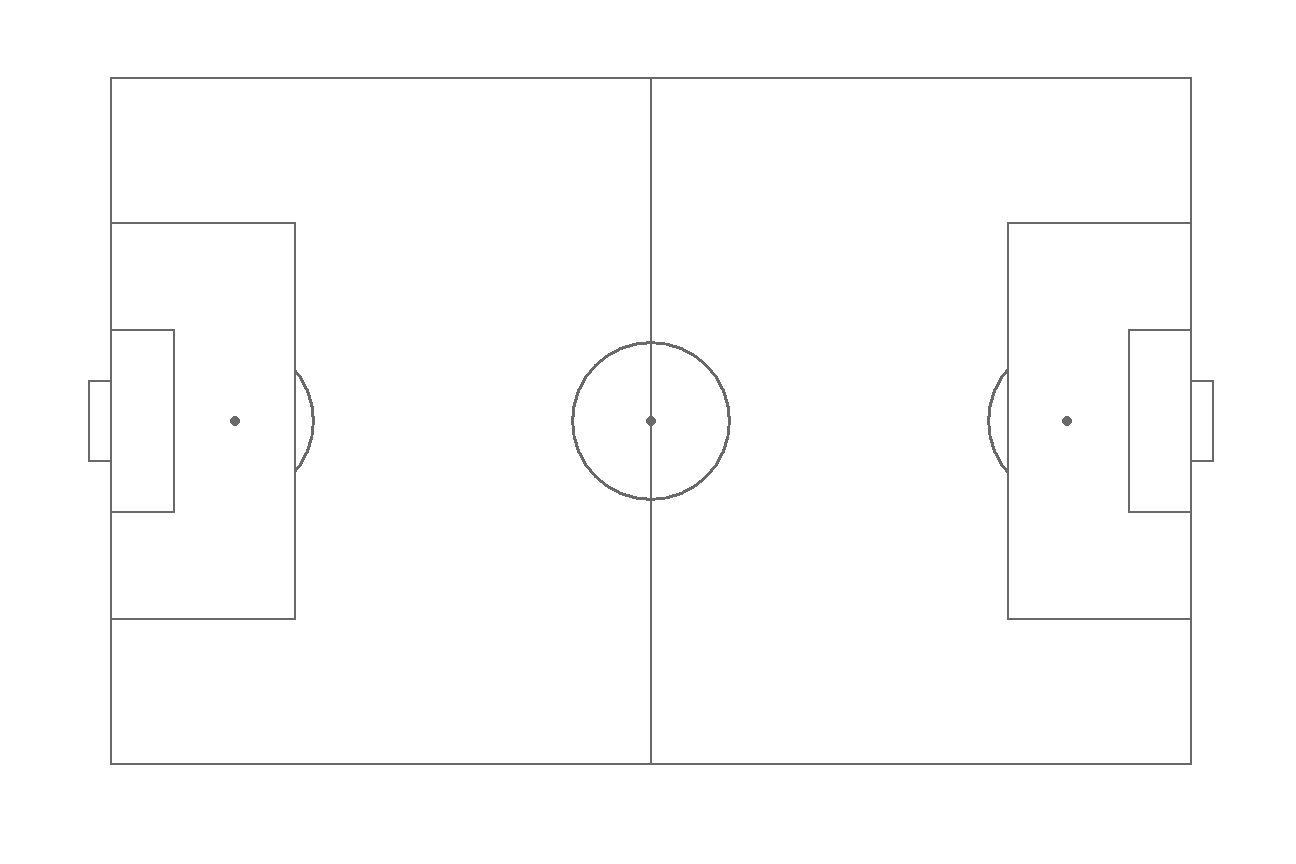## Pitch color

By default the pitch is white with gray lines, but you can customize all the colors. The `colour` argument allows modifying the color of the lines while the `fill` argument modifies the color of the pitch. If you want to change the color of the rest of the plot modify the `panel.background` component of the `theme` function with an `element_rect` as follows.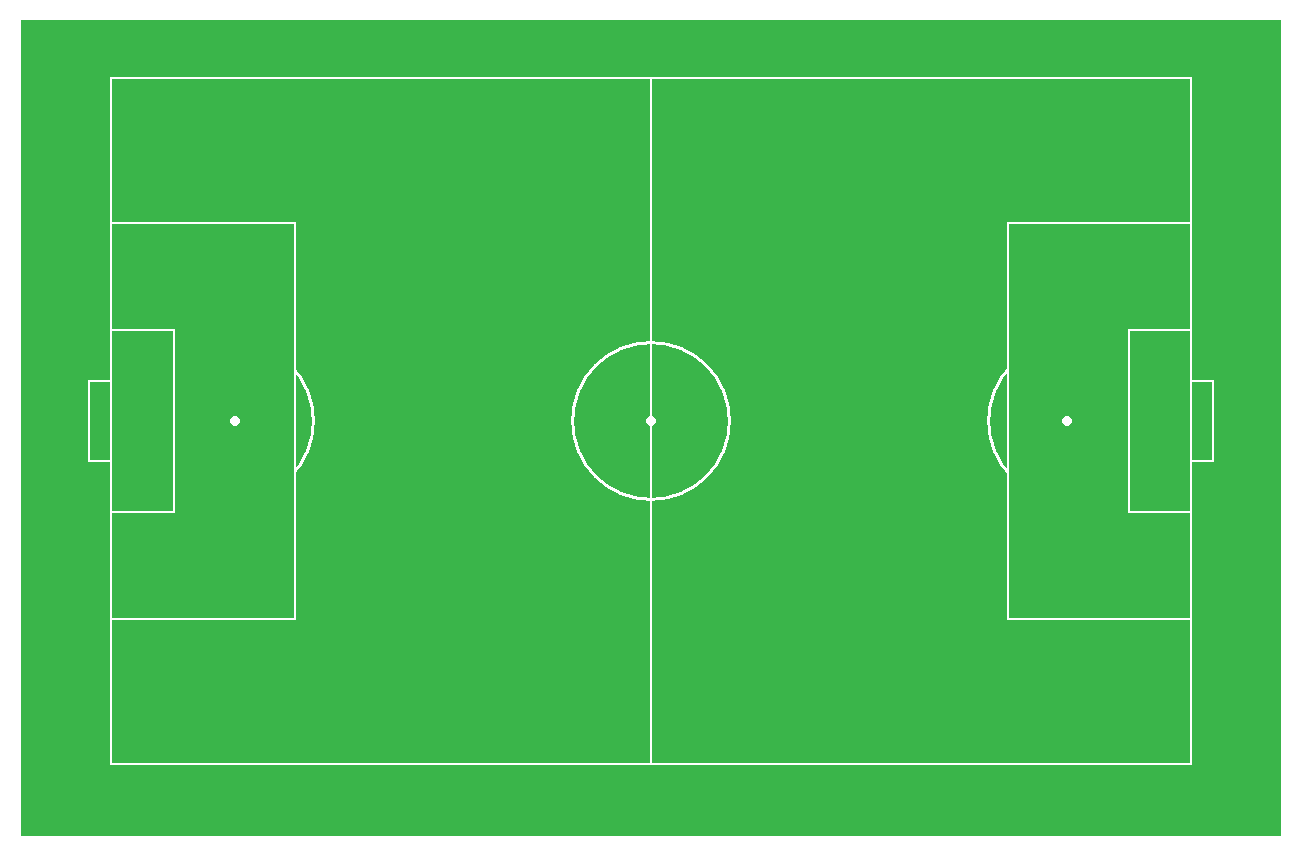``````library(ggplot2)
library(ggsoccer)

ggplot() +
annotate_pitch(colour = "white",
fill = "#3ab54a") +
theme_pitch() +
theme(panel.background = element_rect(fill = "#3ab54a"))``````

## Shot map

You can pass a data frame to create a shot map. Note that the pitch goes from 0 to 100 on both axes. You can also crop the pitch setting axes limits as in any other plot made with ggplot2.

``````library(ggplot2)
library(ggsoccer)

# Data
set.seed(1)
df <- data.frame(x = rnorm(20, 80, 10),
y = rnorm(20, 50, 20),
Shot = sample(c("In", "Out"),
40, replace = TRUE))

ggplot(df) +
annotate_pitch(colour = "white",
fill = "#3ab54a") +
geom_point(aes(x = x, y = y, fill = Shot),
shape = 21,
size = 4) +
coord_cartesian(xlim = c(45, 105))+
theme_pitch() +
theme(panel.background = element_rect(fill = "#3ab54a"))``````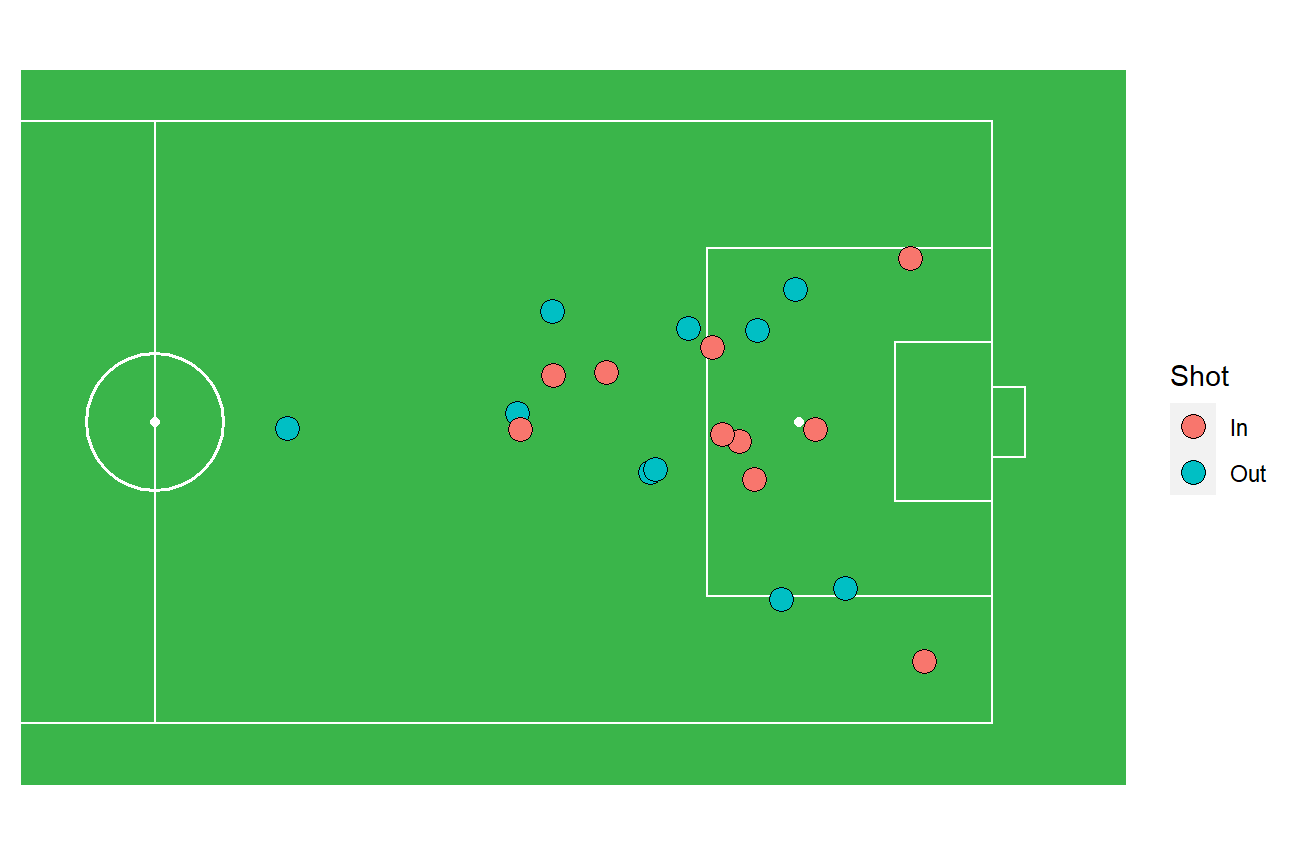## Pass map

Similarly to creating a shot map you can also create a pass map but specifying all the coordinates of the arrows on your data frame and using `geom_segment` to create the arrows.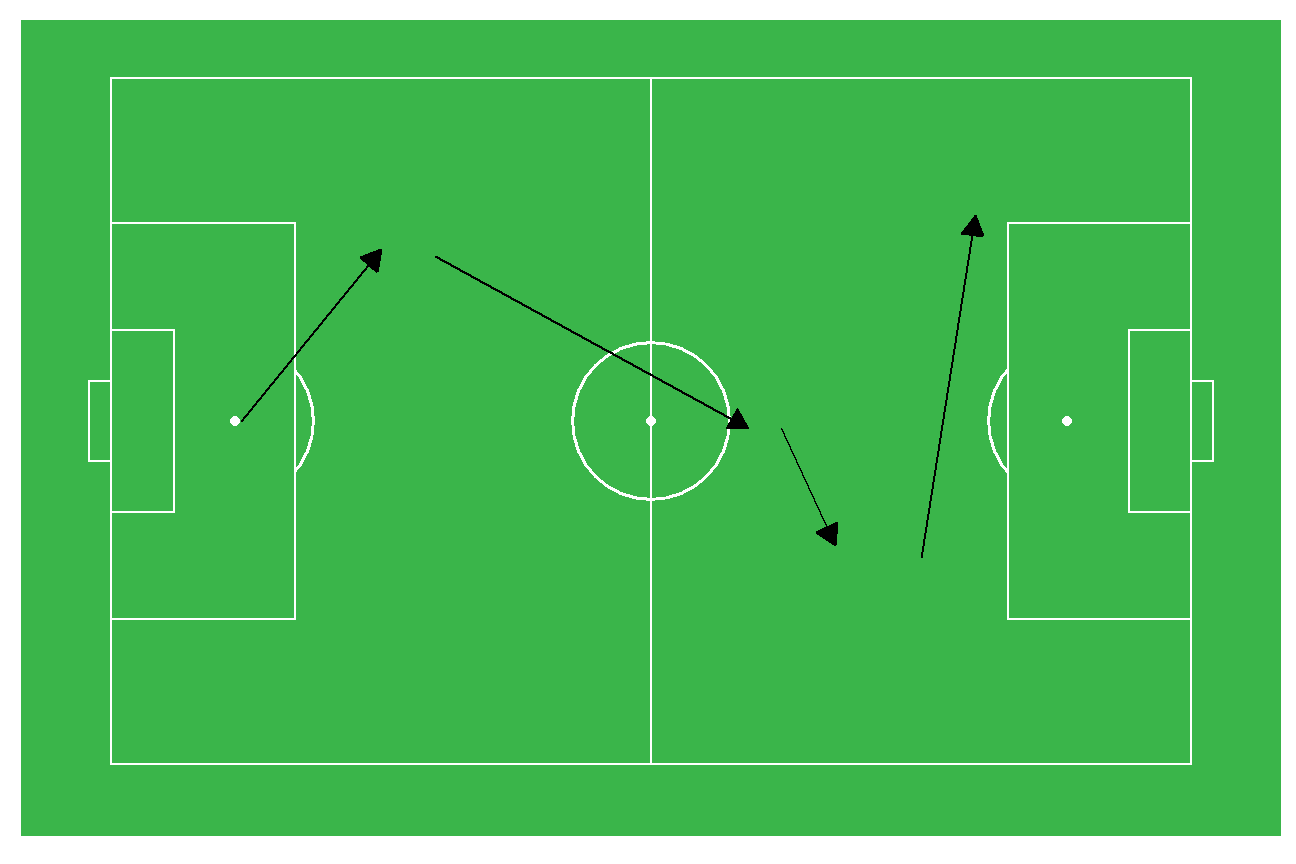``````library(ggplot2)
library(ggsoccer)

# Data
pass <- data.frame(x1 = c(12, 30, 62, 75),
x2 = c(25, 59, 67, 80),
y1 = c(50, 74, 49, 30),
y2 = c(75, 49, 32, 80))

ggplot(pass) +
annotate_pitch(colour = "white",
fill = "#3ab54a") +
geom_segment(aes(x = x1, y = y1, xend = x2, yend = y2),
arrow = arrow(length = unit(0.3, "cm"),
type = "closed")) +
theme_pitch() +
theme(panel.background = element_rect(fill = "#3ab54a"))``````

## Pitch dimensions

The dimensions of the pitch can be modified to fit your needs. Create a list with the corresponding dimensions of each element and pass it to the `annotate_pitch` function.

``````library(ggplot2)
library(ggsoccer)

dimensions <- list(
length = 50,
width = 40,
penalty_box_length = 10,
penalty_box_width = 20,
six_yard_box_length = 2,
six_yard_box_width = 8,
penalty_spot_distance = 7,
goal_width = 6,
origin_x = 0,
origin_y = 0)

ggplot() +
annotate_pitch(dimensions = dimensions) +
theme_pitch()``````